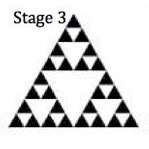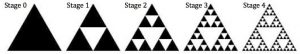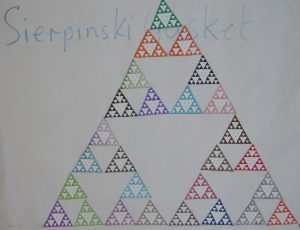Each student should have a stage 3 Sierpinski triangle of edge length about 6.5 cm and a colouring pen, and should colour their triangle as shown by the black filling in the small diagram.

The class will make poster of a stage 6 Sierpinski triangle using 27 of the triangles that the students have coloured in. You can see how, at each stage, 3 triangles are used to make up the Sierpinski triangle at the next stage.

But you have to imagine shrinking the triangles back to the stage 0 size each time.This fractal is the result of repeating the same process infinitely often. The triangles in our poster just help us to  understand the structure of the fractal. Imagine the process going on for ever making more and more and more triangular holes in the gasket.Here is a poster made by a class of a stage 6 Sierpinski triangle.

If you want to extend the 30-minute lesson, and if you have enough time and space, if you have a big class, or if several classes combine their efforts, you might make a stage 7 poster with 81 triangles or even a stage 8 poster with 243 triangles.

NOTES FOR TEACHERS OF DIFFERENT AGE GROUPS

Click here for Notes for Teachers of Upper Primary and Secondary students.

Click here for Notes for Teachers of Lower Primary and Support for Special Needs

Ages 5 to 7

Comparing Pyramids for young learners

Counting, Colours and Triangles for young learners

Ages 7 to 11

Make a von Koch Fractal Poster

Pascal’s Triangle – Number and Fractal Patterns – Multiples

Make a Sierpinski Tetrahedron with toothpicks or newspaper sticks

Make a Christmas Sierpinski Tetrahedron Tree

Ages 11 to 16

Global Maths Lesson – The World We Share – Bar charts

Global Maths Lesson – The World We Share – Pie charts

Pascal’s Triangle – Number and Fractal Patterns – Multiples

Make a von Koch Fractal Poster

The Squareflake Fractal

Sierpinski Triangle – follow-up questions and answers on Number Patterns, Lengths, Areas and Fractions

Sierpinski Tetrahedron – Number and Shape Patterns

Make a Sierpinski Tetrahedron with toothpicks or newspaper sticks

Make a Christmas Sierpinski Tetrahedron Tree

Order to Randomness Download a free booklet giving projects on Visualising Graphs, Really Long Curves, Reasoning and Proof, The Mandelbrot Set, Chaos

Apollonian Circles

Ages 16+

Global Maths Lesson – The World We Share – Pie charts

The Squareflake Fractal

Pascal’s Triangle – Number and Fractal Patterns – Multiples and the Binomial Theorem

Sierpinski Triangle – follow-up questions and answers on Number Patterns, Lengths, Areas and Fractions

Sierpinski Tetrahedron – Number and Shape Patterns

The von Koch Curve – sequences and sums of geometric series

The Chaos Game

Apollonian Circles